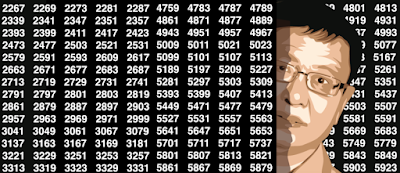## Sunday, September 8, 2019

### Two New Deep Conjectures in Probabilistic Number Theory

The material discussed here is also of interest to machine learning, AI, big data, and data science practitioners, as much of the work is based on heavy data processing, algorithms, efficient coding, testing, and experimentation. Also, it's not just two new conjectures, but paths and suggestions to solve these problems. The last section contains a few new, original exercises, some with solutions, and may be useful to students, researchers, and instructors offering math and statistics classes at the college level: they range from easy to very difficult. Some great probability theorems are also discussed, in layman's terms: see section 1.2.
The two deep conjectures highlighted in this article (conjectures B and C) are related to the digit distribution of well known math constants such as Pi or log 2, with an emphasis on binary digits of SQRT(2). This is an old problem, one of the most famous ones in mathematics, still unsolved today.
A Strange Recursive Formula
• Conjecture A
• A deeper result
• Conjecture B
• Connection to the Berry-Esseen theorem
• Potential path to solving this problem
Potential Solution Based on Special Rational Number Sequences
• Interesting statistical result
• Conjecture C
• Another curious statistical result
Exercises